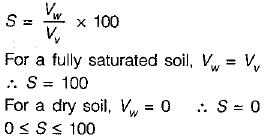Courses

# Properties Of Soil - 3

## 20 Questions MCQ Test Topicwise Question Bank for GATE Civil Engineering | Properties Of Soil - 3

Description
This mock test of Properties Of Soil - 3 for Civil Engineering (CE) helps you for every Civil Engineering (CE) entrance exam. This contains 20 Multiple Choice Questions for Civil Engineering (CE) Properties Of Soil - 3 (mcq) to study with solutions a complete question bank. The solved questions answers in this Properties Of Soil - 3 quiz give you a good mix of easy questions and tough questions. Civil Engineering (CE) students definitely take this Properties Of Soil - 3 exercise for a better result in the exam. You can find other Properties Of Soil - 3 extra questions, long questions & short questions for Civil Engineering (CE) on EduRev as well by searching above.
QUESTION: 1

Solution:
QUESTION: 2

### An undisturbed soil sample has a plastic limit of 25%, a natural moisture content of 40% and liquidity index of 50%, Its liquid limit in % will be

Solution: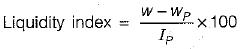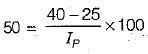⇒ Ip = 30%
Also Ip = wl - wp
⇒ Wl = 30 + 25 = 55%

QUESTION: 3

### A well graded soil has a coefficient of curvature between

Solution:
QUESTION: 4

The ratio of the undisturbed shear strength to the remoulded shear strength in cohesive soil under undrained conditions is

Solution: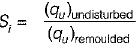at unaltered water condition i.e. at undrained condition. Remoulded shear strength is less than undisturbed shear strength.

QUESTION: 5

Number of phases in soil mass is:

Solution:

Soil has three phase representation as follows,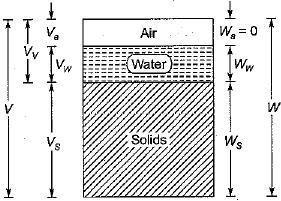QUESTION: 6

Which of the following is a measure of particle size range?

Solution:

The uniformity of a soil is expressed qualitatively by a term known as uniformity coefficient, Cu, given by,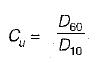The larger the numerical value of Cu, the more is the range of particles.

QUESTION: 7

The ratio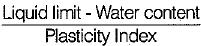for a soil mass is called:

Solution:

The consistency index indicates the consistency (firmness) of a soil. It shows the nearness of the water content of the soil to its plastic limit. A soil with a consistency index of zero is at the liquid limit. It is extremely soft and has negligible shear strength. On the other hand, a soil at a water content equal to the plastic limit has a consistency index of 100%, indicating that the soil is relatively firm.

QUESTION: 8

The principle involved in the relation
γsub. = γsat - γw is

Solution:

When the soil exists below Water, it is in a submerged condition. When a volume  V of soil is sub merged in water, then according to Archimede’s principle it displaces an equal volume of water. Thus the net mass of soil when submerged is reduced.

QUESTION: 9

The uniformity coefficient of soil is given as

Solution:

Uniformity of a soil is expressed qualitatively by uniformity coefficient. The larger the numerical value of Cu more is the ranges of particles.

QUESTION: 10

Given for a soil sample:
Degree of saturation = 90%
Specific gravity of soil grains = 270
Void ratio = 0.30
The water content of the sample is

Solution: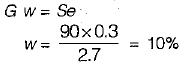QUESTION: 11

For distinguishing clays from silts in the field, a moist soil is rolled into a thread of 3 mm diameter. This test will indicate the

Solution:

The test described is called 'rolling test' or ‘toughness test’ and indicates the toughness.

QUESTION: 12

Which one of the following is the water content of the mixed soil made from 1 kg of soil (say A) with water content of 100% and 1 kg of soil (say B) with water content of 50%?

Solution:

Water content,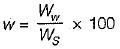Total weight,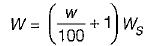Weight of solids in soil A,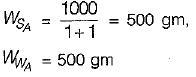Weight of solids in soil B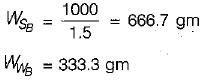In mixed soil,
Ww = 500 + 333.3 = 833.3 gm
Ws = 500 + 666.7 = 1166.7 gm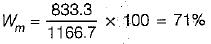QUESTION: 13

Which one of the following gives the correct decreasing order of the densities of a soil sample?

Solution:

We know that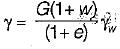For dry soil, w = 0;
For saturated soil, w = ws
and for Wet soil 0 < w < ws
Thus, dry density < wet density < saturated density.
Now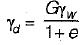and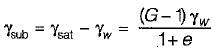Thus submerged density < dry density

QUESTION: 14

Match List-I (Property of soil) with List-ll (Laboratory equipment) and select the correct answer using the codes given below
List-I
A. Grain size
B. Specific gravity
C. Coefficient of permeability
D. Cohesion

List-ll
1. Pycnometer
2. Permeameter
3. Vane shear apparatus
4. Pipette
Codes: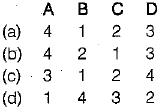Solution:
QUESTION: 15

For a given soil sample,
If Cc = 1.0 and Cu = 4.0, then the value of D30/D10 would be

Solution: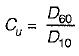and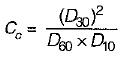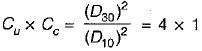⇒ D30/D10 = 2

QUESTION: 16

At liquid limit, all soils possess

Solution:
QUESTION: 17

Which of the following methods is most accurate for the determination of the water content of soil?

Solution:
QUESTION: 18

A pycnometer is used to determine

Solution:
QUESTION: 19

In hydrometer analysis for a soil mass

Solution:

R = Rh' + cm ± Ct - Cd
Rh' = Observed hydrometer reading
Cm = Meniscus correction
Ct - Temperature correction
Cd = Dispersive agent correction

QUESTION: 20

Valid range for S, the degree of saturation of soil in percentage is

Solution:

Degree of saturation represents the portion of volume of voids which is filled with water i.e.